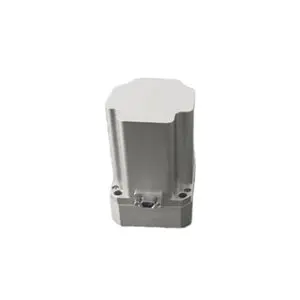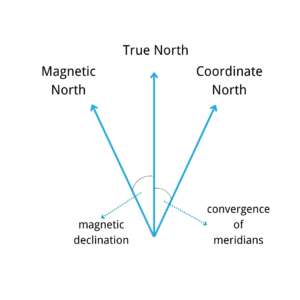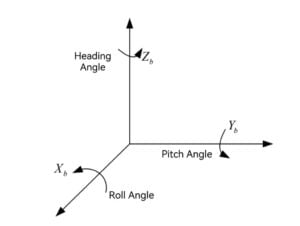Application

# What is North-seeking related coordinate system?True North, Magnetic North, and Coordinate North are the three most commonly used north. True north is the tangent direction of the earth’s north-south line, which is fixed and does not change. Magnetic north is the end point of all magnetic longitude lines in the direction of the earth’s magnetic force, which varies with the change of the magnetic field. Coordinate North is a zonal projection of true North, which coincides with true North only at the central meridian, and deviation of the Meridian Convergence Angle will occur at the non-central meridian. The three have the relationship as shown in the figure.Inertial navigation is carried out in inertial space, which is composed of a series of orthogonal coordinate systems. There are several coordinate systems commonly used in the measurement of inertial sensor parts.

Inertial coordinate system(OXiYiZi)

Fixed with inertial space, the origin does not move and no rotation, absolutely stationary. The origin of the coordinates is usually the center of the earth, The Xi and Yi axis are in the Earth’s equatorial plane, and the Zi axis is parallel to the Earth’s axis and points to the North Pole.

It is also known as the northeast celestial coordinate system, and the origin of the coordinates is the point of the navigation system.The Xn and Yn axis point due east and north respectively, while the Zn axis points to the local vertical.

Carrier coordinate system(OXbYbZb)

There are different forms of the coordinate system depending on the vector. The carrier coordinate system records the attitude information of the carrier, and the Centroid of the carrier is the origin. Xb, Yb, and Zb point to the front, right, and up directions of the system.

There is also a certain relationship between coordinate systems. In an ideal state, there is no attitude error after the horizontal alignment of the gyroscope in the navigation system, and the navigation coordinate system and the carrier coordinate system can be regarded as a coincidence. The position relation between the axes of the b system is the attitude Angle, which is respectively the heading Angle (φ), the pitch Angle (θ), and the roll Angle (γ).φ, γ refers to the Angle obtained by clockwise rotation of the carrier around the Zb and Yb axes respectively, θ refers to the Angle obtained by counterclockwise rotation of the carrier around the Xb axis, as shown in the figure.The navigation coordinate system first rotates about the Zn axis, then rotates θ about the new X axis, and finally rotates γ about the new Y axis, and then coincides with the carrier coordinate system.

We have our own north seeker with a variety of precision， which is designed and produced by Ericco team. If you are interested, please feel free to contact us.